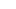Hiak Education Services Pte Ltd

#11-04 Singapore 449408# June 2018 Holiday Programme

April 25, 2018

[LIMITED SLOTS ONLY]

Our annual highly reviewed June Holiday Programme is launching! Secure your slots now! Parents have reviewed that it strengthens their child’s math or even regain confidence in heuristic questions JUST IN 3 DAYS!

This June, PSLEMath has designed an intensive course specially for P2-P6 students! This intensive 3 days course focuses on the heuristic questions that have been specially chosen by our Math Specialist to prepare them for their end of year exams! This Programme also acts as a strong recap for students who had face difficulty during the first semester.

Primary 2

Number of lessons: 3 x 2 hours lessons

Fees: \$160

Lesson 1: Whole number

Comparison of quantity

Comparison of quantity (3 elements)

Comparison of quantity (Age)

Comparison of units

Lesson 2: Whole number

Part and whole

Working backwards

Lesson 3: Measurements

Length (Comparison of length)

Length (Direct application)

Length (Indirect application)

Length (Repeat application)

Mass (Total concept)

Primary 3

Number of lessons: 3 x 2 hours lessons

Fees: \$200

Lesson 1: Whole number

Time basics (Start time, End time & duration)

Money (Equal concept)

Money (Equal elimination)

Lesson 2: Whole number

Whole numbers - Assumption method

Whole numbers - Comparison of quantities and units

Whole numbers - Unchanged total

Whole numbers - Unchanged difference

Lesson 3: Whole numbers

Whole numbers - Shortage and surplus

Whole numbers - Stacking model

Whole numbers - Quantity and value

Whole numbers - Interval concept

Primary 4

Number of lessons: 3 x 2 hours lessons

Fees: \$235

Lesson 1: Whole number

Whole numbers - Assumption method

Whole numbers - Shortage and surplus

Whole numbers - Total concept

Whole numbers - Comparison of quantities

Whole numbers - Comparison of units

Whole numbers - Comparison of quantities & units

Lesson 2: Fractions

Fractions - Basics

Fractions - Comparison of quantities and units

Fractions - Stacking model

Fractions - Part and whole

Lesson 3: Geometry

Geometry - Parallel and perpendicular lines

Geometry - Angles

Geometry - Angles in squares and rectangles

Geometry - 4-point compass

Geometry - Area and perimeter

Primary 5

Number of lessons: 4 x 2 hours lessons

Fees: \$335

Lesson 1: Whole number

Approximation & Estimation

Factors and multiples

Using Units to Substitute a Value

Venn Diagram

Total concept

Grouping - Ignore remainder

Grouping - Consider remainder

Difference concept

Lesson 2: Fractions

Branching out - Proportion of quantity

Branching out - Proportion of quantity with additional value

Branching out - Unknown remainder

Branching out- Unitary method

Lesson 3: Ratios

Identifying Common Base of Comparison - Group

Identifying Common Base of Comparison - Total

Equivalent Proportion

Quantity and Value

Lesson 4: Ratios

Unchanged total

Unchanged difference

Inward/Outward 1 Unchanged

Inward/Outward All Changed

Primary 6

Number of lessons: 4 x 2 hours lessons

Fees: \$350

Lesson 1: Fraction, Percentage, Decimals and Ratio

Identifying Common Base of Comparison - Group

Identifying Common Base of Comparison - Total

Equivalent Proportion - Direct Comparison

Equivalent Proportion - Indirect Comparison

Quantity & Value Concept - Value Given in Total

Lesson 2: Fractions

Branching Out - Proportion of Quantity Only

Branching Out - Proportion of Quantity with Additional Value

Branching Out - Unknown Remainder

Branching Out - Involves Ratio

Branching Out - Unitary Method

Lesson 3: Proportion Transfer Concept

Proportion Transfer Concept - Working Forward

Proportion Transfer Concept - Working Backward

Proportion Transfer Concept - Finding Proportion of Difference

Difference Concept

Lesson 4: Others

Volume

Circle

Geometry

Speed

###### Featured Posts

Factors and Multiples

March 17, 20161/1